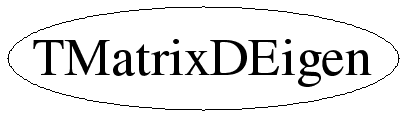# class TMatrixDEigen

```
TMatrixDEigen

Eigenvalues and eigenvectors of a real matrix.

If A is not symmetric, then the eigenvalue matrix D is block
diagonal with the real eigenvalues in 1-by-1 blocks and any complex
eigenvalues, a + i*b, in 2-by-2 blocks, [a, b; -b, a].  That is, if
the complex eigenvalues look like

u + iv     .        .          .      .    .
u - iv     .          .      .
a + ib       .
.        a - ib
.        .          .      x
.        .        .          .      .    y

then D looks like

u        v        .          .      .    .
-v        u        .          .      .    .
a          b
-b          a
.        .          .      x
.        .        .          .      .    y

This keeps V a real matrix in both symmetric and non-symmetric
cases, and A*V = V*D.

```

## Function Members (Methods)

public:
 TMatrixDEigen() TMatrixDEigen(const TMatrixD& a) TMatrixDEigen(const TMatrixDEigen& another) virtual ~TMatrixDEigen() static TClass* Class() const TMatrixD GetEigenValues() const const TVectorD& GetEigenValuesIm() const const TVectorD& GetEigenValuesRe() const const TMatrixD& GetEigenVectors() const virtual TClass* IsA() const TMatrixDEigen& operator=(const TMatrixDEigen& source) virtual void ShowMembers(TMemberInspector& insp, char* parent) virtual void Streamer(TBuffer& b) void StreamerNVirtual(TBuffer& b)
protected:
 static void MakeHessenBerg(TMatrixD& v, TVectorD& ortho, TMatrixD& H) static void MakeSchurr(TMatrixD& v, TVectorD& d, TVectorD& e, TMatrixD& H) static void Sort(TMatrixD& v, TVectorD& d, TVectorD& e)

## Data Members

public:
 enum { kWorkMax };
protected:
 TVectorD fEigenValuesIm Eigen-values TVectorD fEigenValuesRe Eigen-values TMatrixD fEigenVectors Eigen-vectors of matrix

## Class Charts## Function documentation

TMatrixDEigen(const TMatrixD &a)
``` Constructor for eigen-problem of matrix A .
```
TMatrixDEigen(const TMatrixDEigen &another)
``` Copy constructor
```
void MakeHessenBerg(TMatrixD& v, TVectorD& ortho, TMatrixD& H)
``` Nonsymmetric reduction to Hessenberg form.
This is derived from the Algol procedures orthes and ortran, by Martin and Wilkinson,
Handbook for Auto. Comp., Vol.ii-Linear Algebra, and the corresponding
Fortran subroutines in EISPACK.
```
void MakeSchurr(TMatrixD& v, TVectorD& d, TVectorD& e, TMatrixD& H)
``` Nonsymmetric reduction from Hessenberg to real Schur form.
This is derived from the Algol procedure hqr2, by Martin and Wilkinson,
Handbook for Auto. Comp., Vol.ii-Linear Algebra, and the corresponding
Fortran subroutine in EISPACK.
```
void Sort(TMatrixD& v, TVectorD& d, TVectorD& e)
``` Sort eigenvalues and corresponding vectors in descending order of Re^2+Im^2
of the complex eigenvalues .
```
TMatrixDEigen & operator=(const TMatrixDEigen& source)
``` Assignment operator
```
const TMatrixD GetEigenValues()
``` Computes the block diagonal eigenvalue matrix.
If the original matrix A is not symmetric, then the eigenvalue
matrix D is block diagonal with the real eigenvalues in 1-by-1
blocks and any complex eigenvalues,
a + i*b, in 2-by-2 blocks, [a, b; -b, a].
That is, if the complex eigenvalues look like

u + iv     .        .          .      .    .
.      u - iv     .          .      .    .
.        .      a + ib       .      .    .
.        .        .        a - ib   .    .
.        .        .          .      x    .
.        .        .          .      .    y

then D looks like

u        v        .          .      .    .
-v        u        .          .      .    .
.        .        a          b      .    .
.        .       -b          a      .    .
.        .        .          .      x    .
.        .        .          .      .    y

This keeps V a real matrix in both symmetric and non-symmetric
cases, and A*V = V*D.

Indexing:
If matrix A has the index/shape (rowLwb,rowUpb,rowLwb,rowUpb)
each eigen-vector must have the shape (rowLwb,rowUpb) .
For convinience, the column index of the eigen-vector matrix
also runs from rowLwb to rowUpb so that the returned matrix
has also index/shape (rowLwb,rowUpb,rowLwb,rowUpb) .

```

`{}`
virtual ~TMatrixDEigen()
`{}`
const TMatrixD & GetEigenVectors()
``` If matrix A has shape (rowLwb,rowUpb,rowLwb,rowUpb), then each eigen-vector
must have an index running between (rowLwb,rowUpb) .
For convenience, the column index of the eigen-vector matrix
also runs from rowLwb to rowUpb so that the returned matrix
has also index/shape (rowLwb,rowUpb,rowLwb,rowUpb) .
The same is true for the eigen-value vectors an matrix .
```
`{ return fEigenVectors; }`
const TVectorD & GetEigenValuesRe()
`{ return fEigenValuesRe; }`
const TVectorD & GetEigenValuesIm()
`{ return fEigenValuesIm; }`

Last update: root/matrix:\$Id: TMatrixDEigen.h 20882 2007-11-19 11:31:26Z rdm \$